# Peterson correspondence

(diff) ← Older revision | Latest revision (diff) | Newer revision → (diff)

A correspondence between two surfaces under which their tangent planes at corresponding points are parallel. It was considered in general form by K.M. Peterson  in connection with the problem of deformation over a principal base. For example, there is a Peterson correspondence between a surface and it spherical image (cf. Spherical map), between a surface and its rotation indicatrix and between adjoint minimal surfaces (cf. Adjoint surface).

If two surfacesand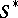in Peterson correspondence have a common parametrization, then their third fundamental forms are equal. The principal net for the asymptotic nets (cf. Asymptotic net) ofandis conjugate (cf. Conjugate net) on each of them. This net is uniquely defined if the asymptotic nets do not have common families of lines, it degenerates if these nets are linked and it becomes undefined if these nets correspond to one another. Corresponding tangents to the lines of the principal net inandare parallel.

If the principal net is taken to be a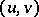coordinate net, then the position vectorsand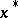ofandare related by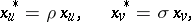where the functionsandsatisfy the system of equationsi.e.anddepend only on the metric of(,andare the coefficients of the first fundamental form). One therefore naturally can apply a Peterson correspondence to a pair of isometric surfacesand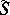: it gives another pair of isometric surfacesand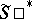with the same normals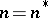and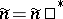, respectively. Also, the rotation diagrams for these surfaces are the same, and the base of the isometry for the new surfaces has the same spherical image as the original ones. For example, a sphere and a surface of constant positive curvature isometric with it correspond under a Peterson correspondence to isometric surfaces with corresponding lines of curvature, so-called Bonnet surfaces.

In particular, if the base of the isometry forandis a principal base, then it remains such. This is the so-called Peterson transformation of a surface deformed over a principal base into a surface of the same type. There is an extension of this transformation to the case of a family of nets .

A special case of a Peterson correspondence is that in which the principal net is a curvature net simultaneously inand. This is called a Combescour correspondence.

If a Peterson correspondence is conformal, then either one of the surfaces is minimal and the other is a sphere (i.e. the Peterson correspondence is a spherical mapping), or the two surfaces are minimal and the conformal mapping vector satisfies the Laplace equation, or the surfaces are similar, or the surfaces are isothermal and are in Combescour correspondence.

There is a multi-dimensional generalization of the Peterson correspondence .

How to Cite This Entry:
Peterson correspondence. Encyclopedia of Mathematics. URL: http://encyclopediaofmath.org/index.php?title=Peterson_correspondence&oldid=14206
This article was adapted from an original article by M.I. Voitsekhovskii (originator), which appeared in Encyclopedia of Mathematics - ISBN 1402006098. See original article